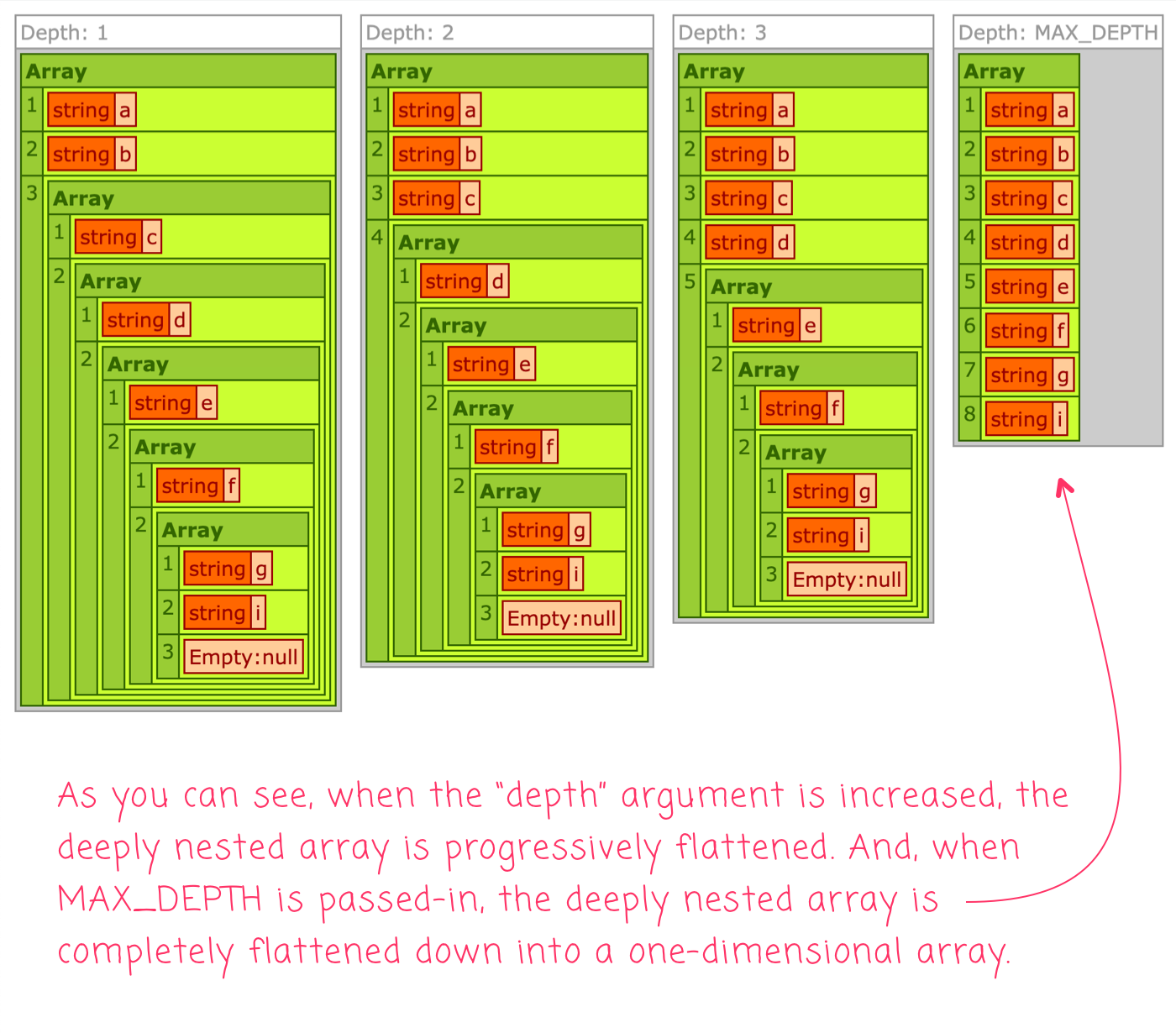# Code Kata: Recursively Flattening A Deep Array In Lucee CFML

By on
Tags:

Yesterday, I looked at flattening an array in ColdFusion. That post was more a look at the available syntax options with a variadic function and less a look at the actual Array flattening algorithm. And, it only flattened to a single depth. As a fast-follow, I wanted to look at what it would take to recursively flatten a deep array, with nested array elements, in Lucee CFML.

The Function signature on this recursive `flatten()` method is based on the `Array.prototype.flat()` method in JavaScript. It takes the Array to flatten and an optional depth that defaults to `1`:

`flatten( values [, depth = 1 ] ) : Array`

When this algorithm encounters a nested Array in the `values` argument, it will look to see if it has reached its maximum depth. If not, it will recursively flatten the nested Array and then merge it into current results. If the algorithm has reached its maximum depth (the "base case"), the nested Array will simply be appended to the results, no further flattening performed.

As a convenience, I'm going to add some metadata to the Function - `MAX_DEPTH` - which is just a large integer that can be passed in as the `depth` argument when the recursion should - for all intents and purposes - go as deep as is needed for the given set of values.

Let's create a deeply nested array and see what happens when we flatten it to different depths:

``````<cfscript>

data = [ "a", nullValue(), [ "b", [ "c", [ "d", [ "e", [ "f", [ "g", "i", nullValue() ] ] ] ] ] ] ];

dump(
var = flatten( data ),
label = "Depth: 1"
);
dump(
var = flatten( data, 2 ),
label = "Depth: 2"
);
dump(
var = flatten( data, 3 ),
label = "Depth: 3"
);
dump(
var = flatten( data, getMetadata( flatten ).MAX_DEPTH ),
label = "Depth: MAX_DEPTH"
);

// ------------------------------------------------------------------------------- //
// ------------------------------------------------------------------------------- //

/**
* I create a new array with all the sub-elements concatenated into it recursively up
* to the given depth. MAX_DEPTH metadata provided as a convenience for "Deep" flatten.
*/
public array function flatten(
required array values,
numeric depth = 1
)
MAX_DEPTH = 2147483647
{

var results = [];

for ( var value in values ) {

// If the array is sparse, skip over any null values during flattening.
if ( isNull( value ) ) {

continue;

}

// RECURSIVE CASE - if the given value is an array and we still have available
// depth to consume, recursively flatten the value and then MERGE its results
// into the current results.
if ( isArray( value ) && depth ) {

results.append( flatten( value, ( depth - 1 ) ), true );

// BASE CASE - end of recursion.
} else {

results.append( value );

}

}

return( results );

}

</cfscript>
``````

As you can see, the `flatten()` method will continue to call itself while there is available `depth`. And, each time the `flatten()` method is recursively called, the `depth` value is decremented. As such, it will eventually recurse down to `0`, fail the recursive test, enter the "base case", and halt recursion.

Test for the Reader: In the given implementation, what would happen if you passed `-1` is as the `depth` argument?

Now, when we run this ColdFusion code, we get the following output:As you can see, as the `depth` argument is increased in value, the resultant array becomes increasingly flat. And, in the final invocation, when we pass-in `MAX_DEPTH` as the `depth` argument, the outcome is a completely flattened, one-dimensional array.

Recursion in fun! ColdFusion is awesome!

Want to use code from this post? Check out the license.